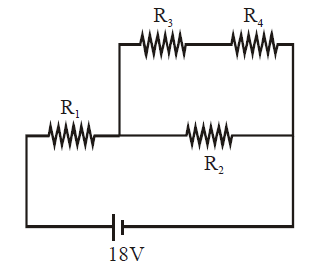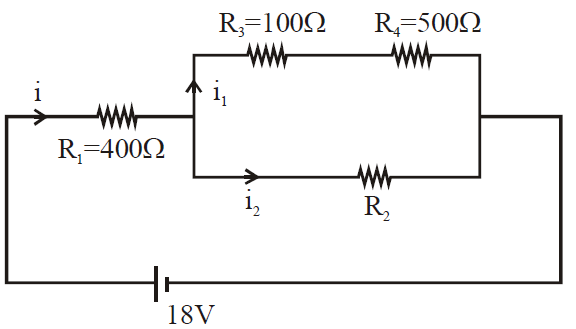# Q. In the given circuit the internal resistance of the 18 V cell is negligible. If $R_1 = 400 \; \Omega , R_3 = 100 \Omega$ and $R_4 = 500 \; \Omega$ and the reading of an ideal voltmeter across $R_4$ is 5V, then the value $R_2$ will be :JEE Main JEE Main 2019

Solution:

## $V_{4} = 5V$ $i_{1} = \frac{V_{4}}{R_{4}} = 0.01 A$ $V_{3} = i_{1} R_{3} = 1V$ $V_{3} +V_{4} = 6V = V_{2}$ $V_{1} + V_{3} + V_{4} = 18 V$ $V_{1} = 12V$ $i = \frac{V_{1} }{R_{1}} = 0.03 Amp .$ $i_{2} = 0.02 Amp V_{2} = 6V$ $R_{2} = \frac{V_{2} }{i_{2}} = \frac{6}{0.02} = 300 \Omega$You must select option to get answer and solution

## 1. The potential to which a conductor is raised, depends on ........

KCET 2005 Electrostatic Potential And Capacitance

## 2. The refractive index of a particular material is 1.67 for blue light, 1.65 for yellow light and 1.63 for red light. The dispersive power of the material is .........

KCET 2004 Electrostatic Potential And Capacitance

## 3. In a permanent magnet at room temperature

KCET 2019 Moving Charges And Magnetism

## 4. An antenna uses electromagnetic waves of frequency 5 MHz. For proper working, the size of the antenna should be

KCET 2019 Communication Systems

## 5. In the following circuit, what are P and Q?

KCET 2019 Semiconductor Electronics: Materials Devices And Simple Circuits

## 6. Two metal plates are separated by 2 cm. The potentials of the plates are − 10 V and + 30 V. The electric field between the two plates is

KCET 2019 Electrostatic Potential And Capacitance

KCET 2019 Nuclei

## 8. For a transistor amplifier, the voltage gain

KCET 2019 Semiconductor Electronics: Materials Devices And Simple Circuits

## 9. “Parsec” is the unit of

BHU 2007 Physical World, Units and Measurements

## 10. “Parsec” is the unit of

BHU 2007 Physical World, Units and Measurements